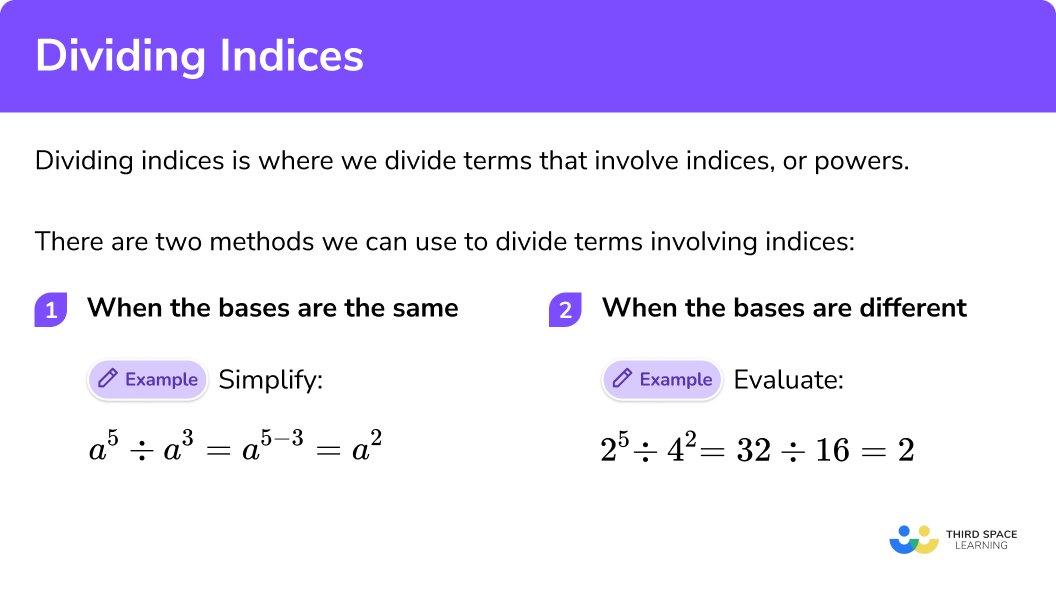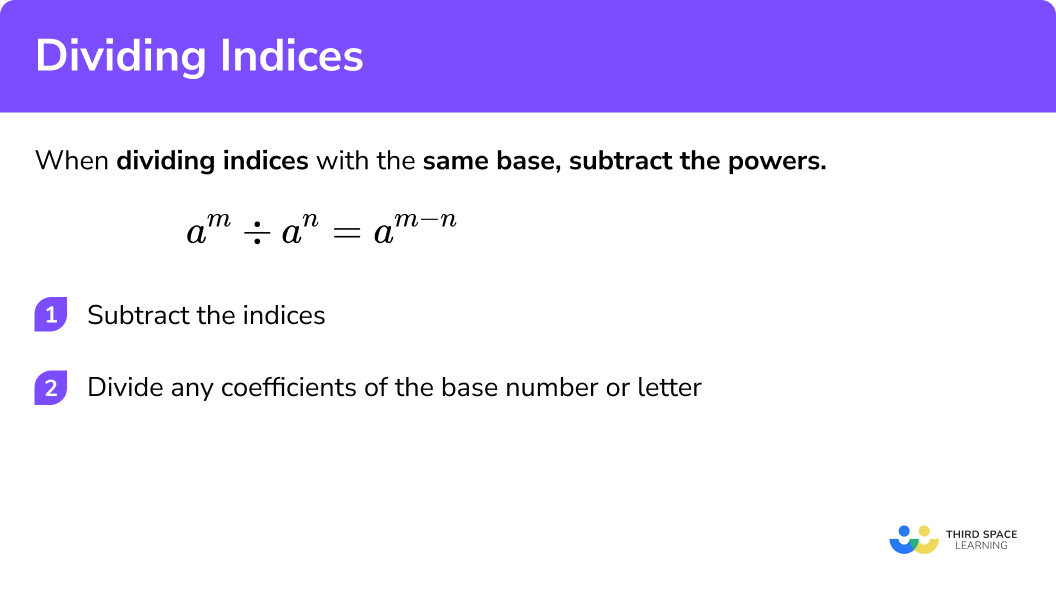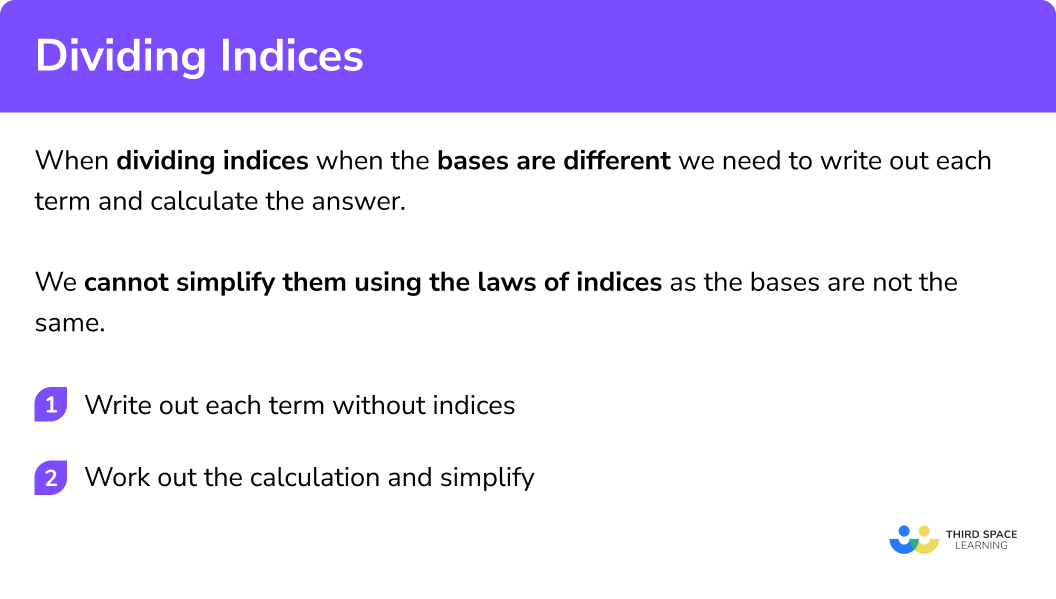GCSE Maths Algebra Law Of Indices

Dividing Indices

# Dividing Indices

Here we will learn about dividing indices including how to use the laws of indices to divide indices and how to divide indices that have different bases.

There are also laws of indices worksheets based on Edexcel, AQA and OCR exam questions, along with further guidance on where to go next if you’re still stuck.

## What is dividing indices?

Dividing indices is where we divide terms that involve indices or powers.

There are two methods we can use to divide terms involving indices.

1 When the bases are the same:

E.g

$a^{5} \div a^{3}=a^{5-3}=a^{2}$

These questions usually ask you to ‘simplify’ the calculation.

2 When the bases are different:

E.g

$2^{5} \div 4^{2}=32 \div 16=2$

These questions usually ask you to ‘evaluate’ (work out) the calculation

### What do we mean by dividing indices?## How to divide indices when the bases are the same

When dividing indices with the same base, subtract the powers.

$a^{m} \div a^{n}=a^{m-n}$

1. Subtract the indices
2. Divide any coefficients of the base number or letter

In order to divide indices when the bases are the same we can use one of the laws of indices.

E.g.

To simplify the following expression:

$8^{7} \div 8^{4}$

We can write out each power in its expanded form:

$8^{7} \div 8^{4}=\frac{8 \times 8 \times 8 \times 8 \times 8 \times 8 \times 8}{8 \times 8 \times 8 \times 8}$

We know that 8 ÷ 8 = 1, so we can keep canceling an 8 on the top with an 8 on bottom until we are left with:

$8 \times 8 \times 8=8^{3}$

We can simplify this process by simplifying 87 ÷ 84 to 87-4 = 83:

$8^{7} \div 8^{4}=8^{7-4}=8^{3}$

The base has stayed the same and we have subtracted the indices.

This is the division law of indices.

### Explain how to divide indices when the bases are the same## Related lessons on laws of indices

Dividing indices is part of our series of lessons to support revision on laws of indices. You may find it helpful to start with the main laws of indices lesson for a summary of what to expect, or use the step by step guides below for further detail on individual topics. Other lessons in this series include:

## Dividing indices examples (with the same base)

### Example 1: no coefficient in front of base

$a^{5} \div a^{2}$

1. Subtract the indices 5 and 2.

$5 – 2 = 3$

So,

$a^{5} \div a^{2}=a^{3}$

### Example 2: with a coefficient in front of base

$21 a^{9} \div 7 a^{2}$

Subtract the indices 9 and 2.

Divide the coefficients 21 and 7.

### Example 3: dividing indices (negative Indices)

$18 a^{-6} \div 9 a^{-4}$

Subtract the powers -6 and the -4.

Divide the coefficients 18 and the 9.

### Example 4: algebraic expressions

$100 a^{6 b} \div-10 a^{-7 b}$

Subtract the indices 6b and -7b.

Divide the coefficients 100 and -10.

### Example 5: with fractional powers

$x^{\frac{4}{5}} \div x^{\frac{2}{3}}$

Subtract the indices ⅘ and ⅔.

### Practice dividing indices questions (with the same base)

x^{9} \div x^{2}

x^{11}x^{4.5}7xx^{7}9-2=7 so

x^{9} \div x^{2} = x^{7}

2. Simplify

12 b^{13} \div 4 b^{7}

3b^{6}8b^{6}3b^{\frac{13}{7}}3^{6}13-7=6

12 \div 4 = 3

12 b^{13} \div 4 b^{7}=3b^{6}

3. Simplify

63^{-2} \div 7 a^{-3}

9a^{\frac{2}{3}}56a9a9a^{-5}-2 – (-3) = -2+3 = 1

63 \div 7=9

63a^{-2} \div 7 a^{-3} = 9a

(When the power is 1 we do not need to write it)

4. Simplify

\frac{24c^{6}d^{3}}{4c^{2}d^{2}}

6cd^{5}20c^{4}d6c^{4}6c^{4}dFor c: 6-2=4

For d: 3-2=1

24 \div 4 = 6

\frac{24c^{6}d^{3}}{4c^{2}d^{2}} = 6c^{4}d

5. Simplify

-100 a^{8 x+2 b} \div 10 a^{3 b}

10 a^{8 x-b}-10 a^{8 x-b}10 a^{8 x+5b}90 a^{8 x-b}8x+2b-3b=8x-b

-100 \div 10 = -10

-100 a^{8 x+2 b} \div 10 a^{3 b}=-10 a^{8 x-b}

6. Simplify

4 x^{\frac{3}{4}} \div 2 x^{\frac{1}{3}}

4 x^{\frac{5}{12}}2 x^{\frac{5}{12}}0.5 x^{\frac{5}{12}}2 x^{\frac{9}{4}}\begin{aligned} \frac{3}{4} – \frac{1}{3} &= \frac{9}{12} – \frac{4}{12}\\\\ &= \frac{5}{12} \end{aligned}

4 \div 2 = 2

4 x^{\frac{3}{4}} \div 2 x^{\frac{1}{3}} = 2 x^{\frac{5}{12}}

## How to divide indices when the bases are different

In order to divide indices when the bases are different we need to write out each term and calculate the answer.

We cannot simplify them using the laws of indices as the bases are not the same.

1 Write out each term without the indices.

2 Work out the calculation and simplify.

E.g.

To evaluate the following expression:

$2^{3} \div 3^{2}$

We need to write each term of the calculation without using index notation:

$2^{3}=2 \times 2 \times 2=8$ $3^{2}=3 \times 3=9$

So we have:

$2^{3} \div 3^{2}=\frac{2 \times 2 \times 2}{3 \times 3}$

Now we need to work out the calculation:

\begin{aligned} 2^{3} \div 3^{2} &=\frac{2 \times 2 \times 2}{3 \times 3} \\ &=\frac{8}{9} \end{aligned}

### Explain how to divide indices when the bases are different## Dividing indices examples (with different bases)

### Example 1: with positive indices

Evaluate:

$4^{2} \div 2^{3}$

1. Write out each term without the indices.

$4^{2} \div 2^{3}=\frac{4 \times 4}{2 \times 2 \times 2}$

2 Work out the calculation and simplify.

\begin{aligned} 4^{2} \div 2^{3} &=\frac{4 \times 4}{2 \times 2 \times 2} \\\\ &=\frac{16}{8} \\\\ &=2 \end{aligned}

### Example 2: with three terms

Evaluate:

$8^{2} \div 2^{4} \div 3^{2}$

Write out each term without the indices.

Work out the calculation and simplify.

### Example 3: with negative powers and fractional powers

This example uses the Negative and Fractional indices. It is a good idea to check our Laws of Indices page for more information before attempting this question.

Evaluate:

$8^{\frac{1}{3}} \div 2^{-2}$

Write out each term without the indices.

Work out the calculation and simplify.

### Practice dividing indices questions (with different bases)

1. Evaluate

5^{2} \div 3^{3}

\frac{25}{27}2^{-1}\frac{25}{81}(\frac{5}{3})^{-1}\begin{aligned} 5^{2} \div 3^{3} &= \frac{5 \times 5}{3 \times 3 \times 3}\\\\ &=\frac{25}{27} \end{aligned}

2. Evaluate

2^{6} \div 4^{2} \div 3^{3}

\frac{5}{30}\frac{2}{27}\frac{4}{27}\frac{8}{27}\begin{aligned} 2^{6} &= 2 \times 2 \times 2 \times 2 \times 2 \times 2 = 64\\\\ 4^{2} &= 4 \times 4 = 16\\\\ 3^{3} &= 3 \times 3 \times 3 = 27 \end{aligned}

\begin{aligned} 2^{6} \div 4^{2} \div 3^{3} &= 64 \div 16 \div 27\\\\ &=\frac{4}{27} \end{aligned}

3. Evaluate

3^{4} \div 2^{-2}

20.25108\frac{81}{\sqrt{2}}324\begin{aligned} 3^{4}&=3 \times 3 \times 3 \times 3=81\\\\ 2^{-2}&=\frac{1}{-2}=\frac{1}{4} \end{aligned}

\begin{aligned} 3^{4} \div 2^{-2} &= 81 \div \frac{1}{4}\\\\ &=81 \times 4\\\\ &=324 \end{aligned}

4. Evaluate

27^{\frac{2}{3}} \div 2^{-2} \div 3^{2}

49\frac{1}{4}\frac{9}{4}\begin{aligned} 27^{\frac{2}{3}}&=9\\\\ 2^{-2}&=\frac{1}{4}\\\\ 3^{2}&=9 \end{aligned}

\begin{aligned} 27^{\frac{2}{3}} \div 2^{-2}\div 3^{2} & = 9 \div \frac{1}{4} \div 9\\\\ &=4 \end{aligned}

### Dividing indices GCSE questions

1. Simplify

x^{2} \div x^{3}

(1 mark)

x^{-1}

(1)

2. Simplify

8 h^{3} m^{6} \div 2 h^{4} m^{2}

(2 marks)

h^{-1} \text { or } m^{4} seen (evidence of subtracting powers)

(1)

4 h^{-1} m^{4}

(1)

3. Simplify

\frac{30 x^{3} y^{-4}}{5 x^{-2} y^{-3}}

(2 marks)

x^{5} \text { or } y^{-1} seen (evidence of subtracting powers)

(1)

6 x^{5} y^{-1}

(1)

### Common misconceptions

• The dividing indices law can only be used for terms with the same base

E.g. We cannot simplify

$a^{4} \div b^{3}$

as the bases are different

• Confusing integer and fractional powers

Raising a term to the power of 2 means we square it

E.g.

$a^{2}=a \times a$

Raising a term to the power of ½ means we find the square root of it

E.g.

$a^{\frac{1}{2}}= \sqrt{a}$

Raising a term to the power of 3 means we cube it

E.g.

$a^{3}=a \times a \times a$

Raising a term to the power of ⅓ means we find the cube root of it

E.g.

$a^{\frac{1}{3}}=\sqrt{a}$

• Indices, powers or exponents

Indices can also be called powers or exponents.

## Learning checklist

You have now learned how to:

• Simplify expressions involving the laws of indices
• Calculate with roots, and with integer and fractional indices

## Still stuck?

Prepare your KS4 students for maths GCSEs success with Third Space Learning. Weekly online one to one GCSE maths revision lessons delivered by expert maths tutors.

Find out more about our GCSE maths tuition programme.